# An Introduction to Bayesian Analysis

## Background

The notes, which are released under a CC BY 4.0 license, were written by Joe Thorley.

## Installation

To install all the required packages execute the following code at the R console.

if(!"drat" %in% installed.packages()[,1])
install.packages("drat")
install.packages("jmbr")

If everything was successful, when you run the following code

library(jmbr)
model <- model("model {
bLambda ~ dlnorm(0,10^-2)
for (i in 1:length(x)) {
x[i]~dpois(bLambda)
}
}")

coef(analyse(model, data = data.frame(x = rpois(100,1))))

you should see something like

# A tibble: 1 x 7
term    estimate     sd zscore lower upper   pvalue
<term>     <dbl>  <dbl>  <dbl> <dbl> <dbl>    <dbl>
1 bLambda    0.858 0.0922   9.33 0.690  1.06 0.000300

During the course you should begin R scripts with

library(dplyr)
library(ggplot2)
library(scales)
library(jmbr)

to load the required packages in the search path and

graphics.off()
rm(list = ls())

to clean up the workspace and close any graphics windows.

# Bayesian and Frequentist Statistical Analysis

Statistical analysis uses one or more probability distributions to provide bounded estimates of parameter values ($$\theta$$) from the data ($$y$$).

There are two primary approaches to statistical analysis: Bayesian and frequentist. As far as a frequentist is concerned the best estimates of $$\theta$$ are those values that maximise the likelihood which is the probability of the data given the estimates, i.e., $$p(y|\theta)$$. A Bayesian on the other hand chooses the values with the highest posterior probability - that is to say the probability of the estimates given the data , i.e., $$p(\theta|y)$$.

## Coin Flips

Consider the case where $$n = 10$$ flips of a coin produce $$y = 3$$ tails. We can model this using a binomial distribution.

$y \sim dbin(\theta, n)$

where $$\theta$$ is the probability of throwing a head.

## Maximum Likelihood

The likelihood for the binomial model is given by the following equation

$p(y|\theta) = {n \choose y} \theta^{y}(1-\theta)^{n-y}$.

The likelihood values for different values of $$\theta$$ are therefore as follows

likelihood <- function(theta, n = 10, y = 3) {
choose(n, y) * theta^y * (1 - theta)^(n - y)
}
theta <- seq(from = 0, to = 1, length.out = 100)

qplot(theta, likelihood(theta), geom = "line", ylab = "Likelihood", xlab = "theta")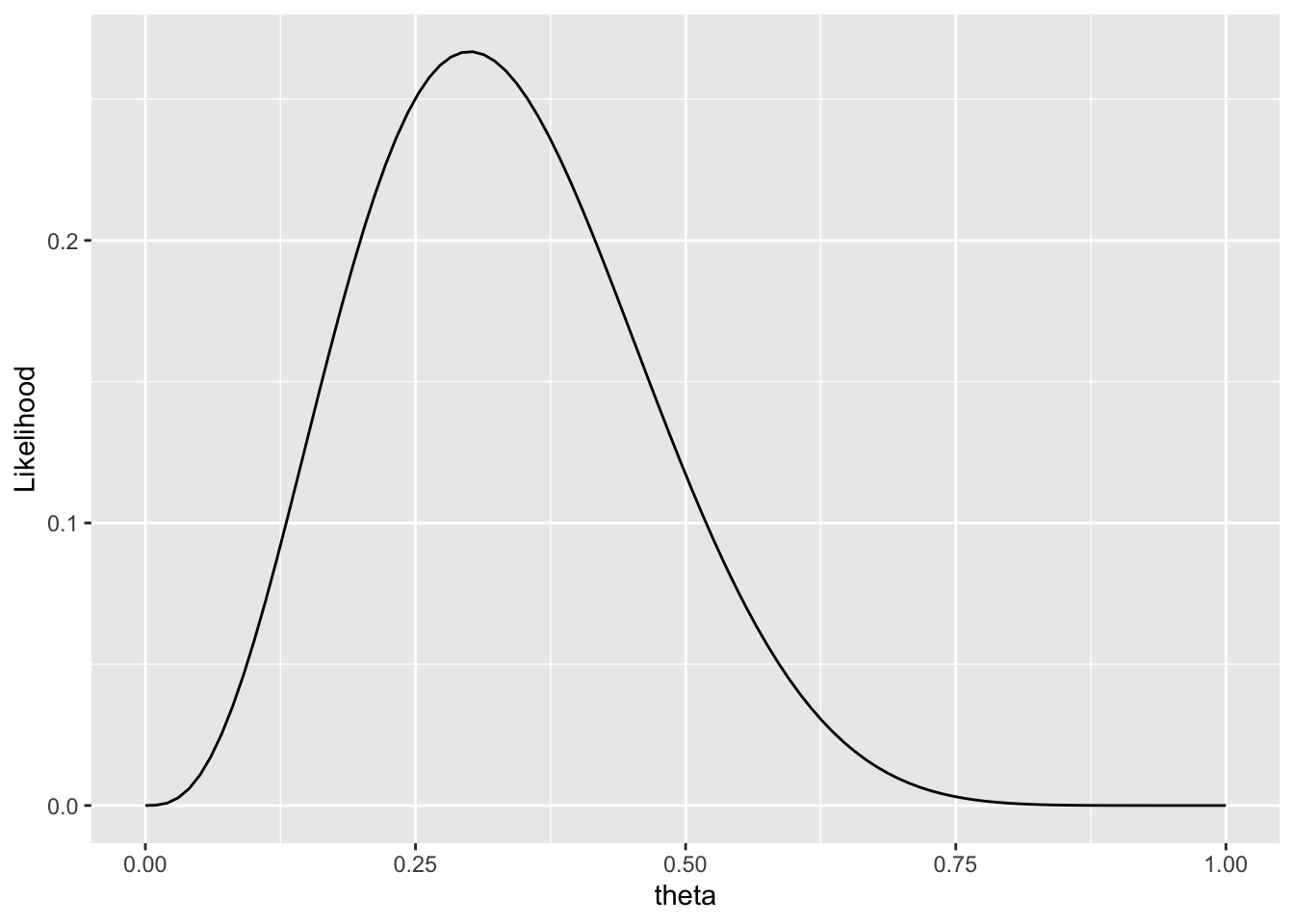The frequentist point estimate ($$\hat{\theta}$$) is the value of $$\theta$$ with the maximum likelihood (ML) value, which in this case is 0.3.

A 95% confidence interval (CI) can then be calculated using the asymptotic normal approximation

$\hat{\theta} \pm 1.96 \frac{1}{\sqrt{I(\hat{\theta})}}$

where $$I(\hat{\theta})$$ is the expected second derivative of the log-likelihood at the estimate. This calculation is based on the assumption that the sample size is of sufficient size that the likelihood is normally distributed.

In the current case,

$I(\hat{\theta}) = \frac{n}{\hat{\theta}(1 - \hat{\theta})}$

which gives a point estimate of 0.3 and lower and upper 95% confidence intervals of 0.02 and 0.58 respectively.

## Posterior Probability

The posterior probability on the other hand is given by Bayes rule which states that

$p(\theta|y) \propto p(y|\theta)p(\theta)$

where $$p(\theta)$$ is the prior probability.

Bayesians consider the necessity to define prior probabilities an advantage because prior information can be incorporated into an analysis while frequentists consider it subjective. In most cases Bayesians use low-information priors which have little to no influence on the posteriors such as a uniform distribution with a lower limit of 0 and an upper limit of 1 for a probability. As it is generally not possible to calculate the posterior probability, the posterior probability distribution is sampled using Markov Chain Monte Carlo (MCMC) algorithms such as Gibbs Sampling.

### Gibbs Sampling

Consider the case where the parameters $$\theta = (\theta_1, \theta_2, \ldots, \theta_k)$$ then Gibbs Sampling proceed as follows

Step 1

Choose starting initial values for $$\theta_1^{(0)}$$ and $$\theta_2^{(0)}$$

Step 2

Sample $$\theta_1^{(1)}$$ from $$p(\theta_1|\theta_2^{(0)}, y)$$

Sample $$\theta_2^{(1)}$$ from $$p(\theta_2|\theta_1^{(1)}, y)$$

Step 3

Iterate step 2 thousands (or millions) of times to obtain a sample from $$p(\theta|y)$$.

Typically this is performed for two or more independent chains.

## JAGS and the BUGS Language

Programming an efficient MCMC algorithm for a particular model is outside the scope of most research projects. Fortunately, JAGS (which stands for Just Another Gibbs Sampler) can take a dataset and a model specified in the simple but flexible BUGS language (which stands for Bayesian Analysis Using Gibbs Sampling) and perform MCMC sampling for us. In order to do this we will use the jmbr package to talk to the standalone JAGS program via the rjags package.

First we need to specify the underlying probability model in the BUGS language and save it as an object of class mb_model.

model1 <- model("model {
theta ~ dunif(0, 1)
y ~ dbin(theta, n)
}") 

then we call JAGS using jmbr (in the default report mode) to generate a total of $$\geq 10^3$$ samples using three chains from $$\theta$$’s posterior probability distribution.

data <- data.frame(n = 10, y = 3)
analysis1 <- analyse(model1, data = data)
## Registered S3 method overwritten by 'rjags':
##   method               from
##   as.mcmc.list.mcarray mcmcr
## # A tibble: 1 x 8
##       n     K nchains niters nthin   ess  rhat converged
##   <int> <int>   <int>  <int> <int> <int> <dbl> <lgl>
## 1     1     1       3   1000     1  1899  1.00 TRUE
plot(analysis1)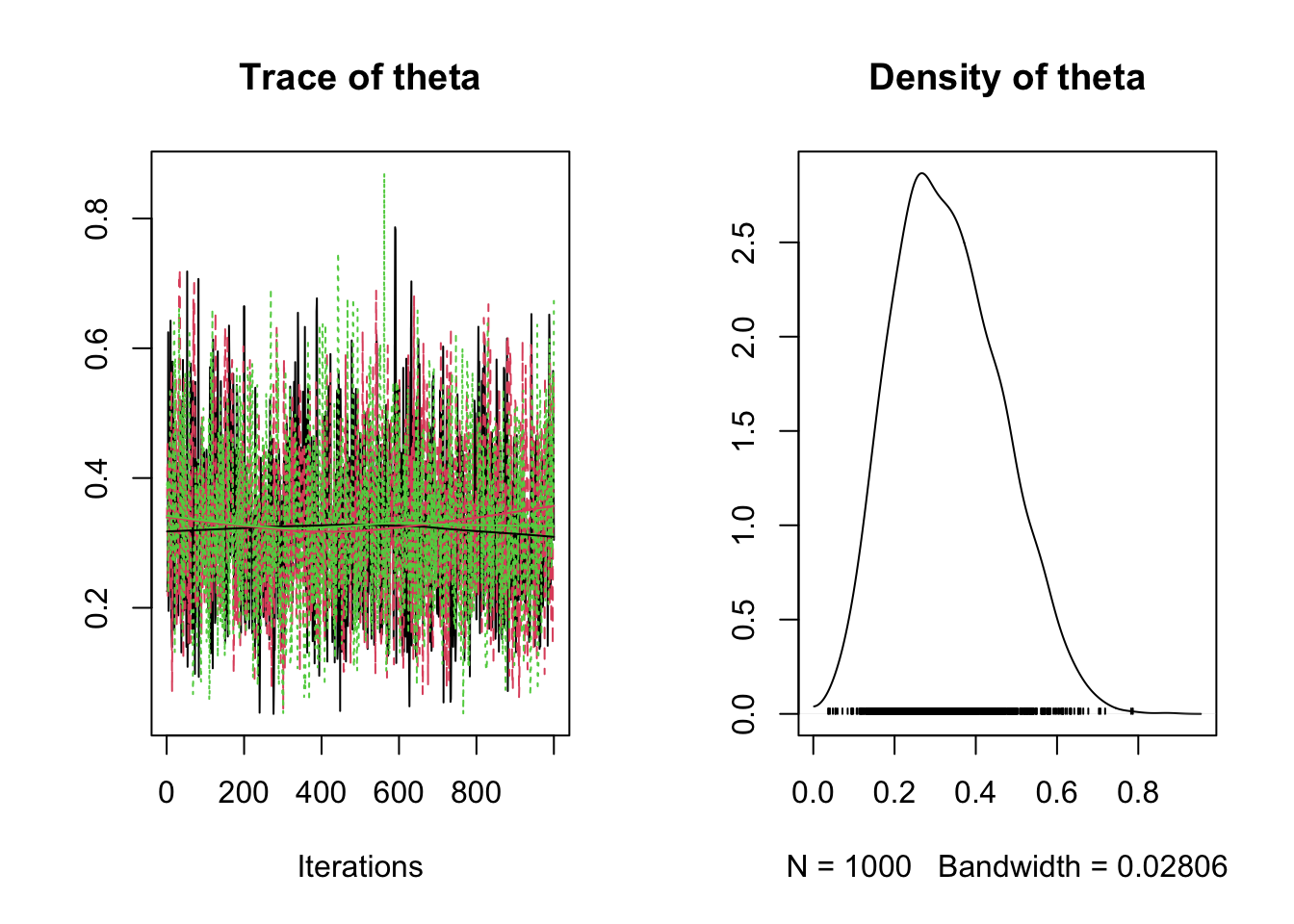coef(analysis1)
## # A tibble: 1 x 7
##   term   estimate    sd zscore lower upper   pvalue
##   <term>    <dbl> <dbl>  <dbl> <dbl> <dbl>    <dbl>
## 1 theta     0.329 0.131   2.57 0.115 0.621 0.000300

The model output indicates that the point estimate (in this case the mean of the samples) is 0.33 and the 95% credible interval (in this case the 2.25th and 97.75th percentiles) is 0.11 to 0.59. The model output also indicates that the posterior probability distribution has a standard deviation (sd) of 0.13. The significance and error values are discussed later.

Exercise 1 Previous studies indicate that the coin was definitely biased towards tails. Modify the prior distribution accordingly and rerun the above model. How does the posterior distribution change?

# Black Cherry Trees

The trees data set in the dataset package provides information on the girth and volume of 31 black cherry trees.

qplot(x = Girth, y = Volume, data = trees)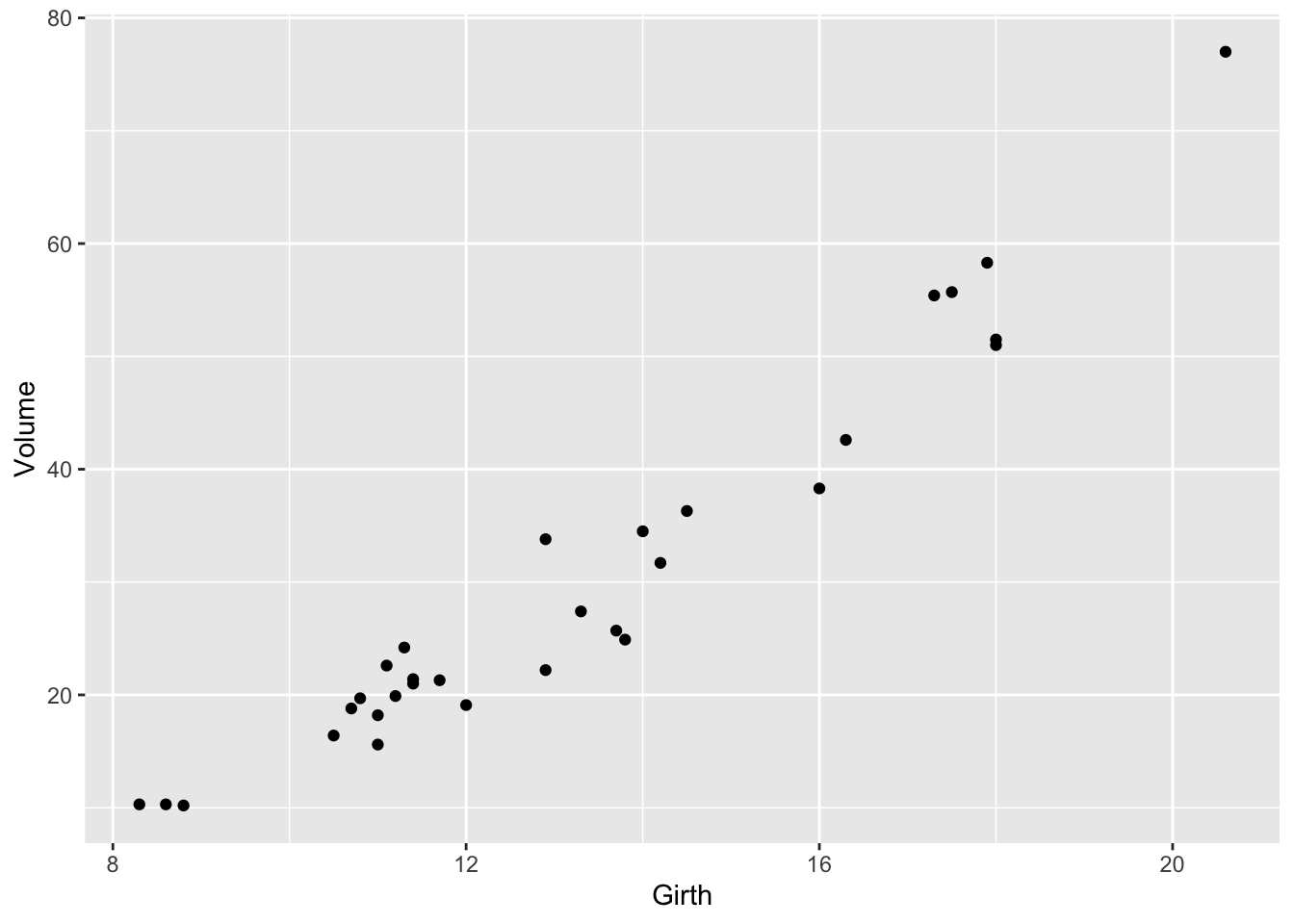Algebraically, the linear regression of Volume against Girth can be defined as follows

$Volume_{i} = \alpha + \beta * Girth_{i} + \epsilon_{i}$

where $$\alpha$$ is the intercept and $$\beta$$ is the slope and the error terms ($$\epsilon_{i}$$) are independently drawn from a normal distribution with an standard deviation of $$\sigma$$.

The model can be defined as follows in the BUGS language where <- indicates a deterministic as opposed to stochastic node (which is indicated by ~).

model1 <- model("model {
alpha ~ dnorm(0, 50^-2)
beta ~ dnorm(0, 10^-2)
sigma ~ dunif(0, 10)

for(i in 1:length(Volume)) {
eMu[i] <- alpha + beta * Girth[i]
Volume[i] ~ dnorm(eMu[i], sigma^-2)
}
}")

## Precision

The standard deviations of the normal distributions are raised to the power of -2 because (for historical reasons) Bayesians quantify variation in terms of the precision ($$\tau$$) as opposed to the variance ($$\sigma^2$$) or standard deviation ($$\sigma$$) where $$\tau = 1/\sigma^2$$.

## Parallel Chains

To reduce the analysis time, MCMC chains can be run on parallel processes. In jmbr this achieved using the registerDoParallel function and by setting the parallel option to TRUE. This only needs to be done once at the start of a session. I add it to my R script header.

library(foreach)
library(doParallel)
## Loading required package: iterators
## Loading required package: parallel
if (getDoParWorkers() == 1)
registerDoParallel(4)

The resultant trace plots and coefficients for the trees analysis are as follows.

data(trees)
analysis1 <- analyse(model1, data = trees)
## # A tibble: 1 x 8
##       n     K nchains niters nthin   ess  rhat converged
##   <int> <int>   <int>  <int> <int> <int> <dbl> <lgl>
## 1    31     3       3   1000     1    81  1.03 FALSE
plot(analysis1, parm = "alpha")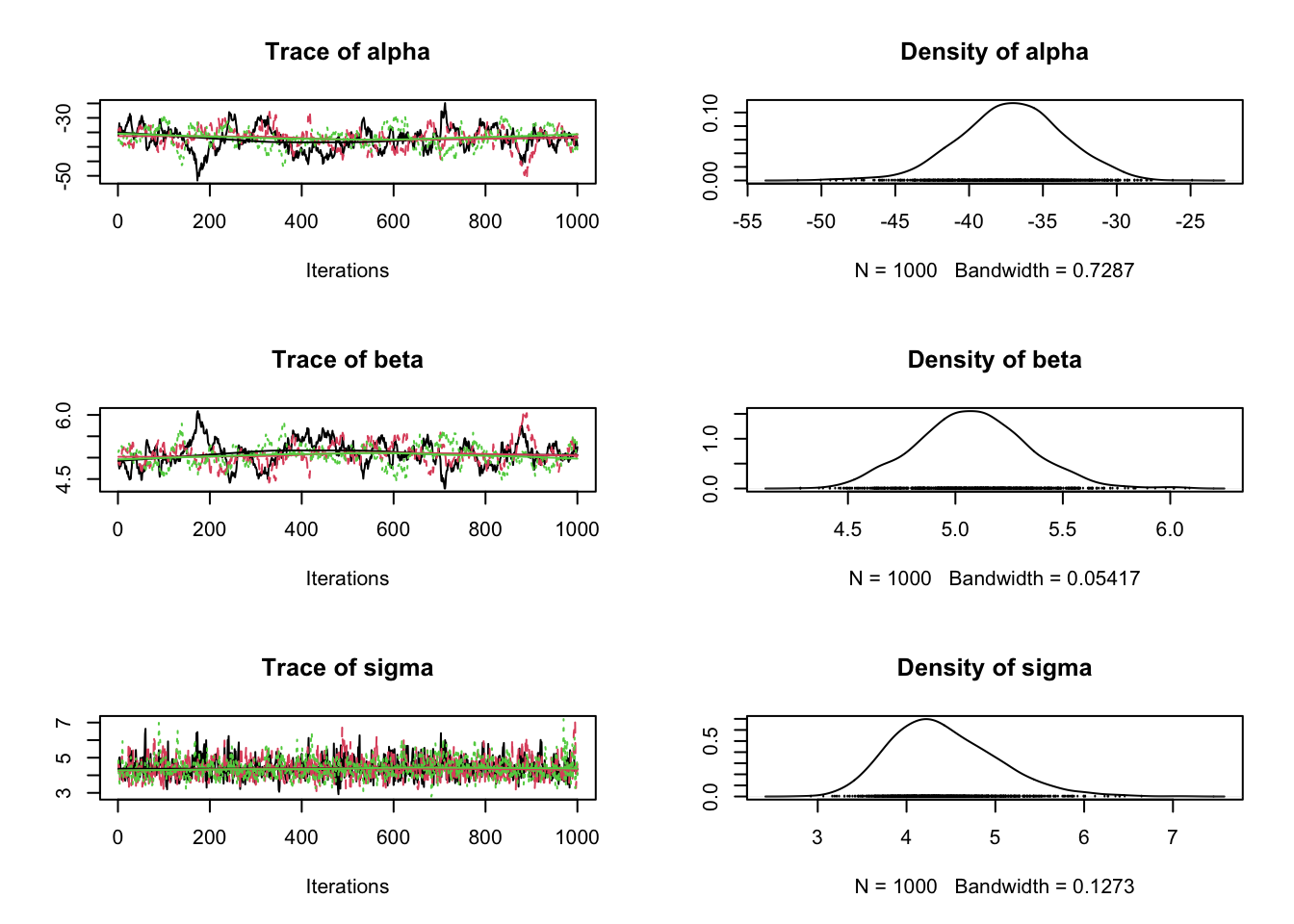plot(analysis1, parm = "beta")plot(analysis1, parm = "sigma")coef(analysis1)
## # A tibble: 3 x 7
##   term   estimate    sd zscore  lower  upper   pvalue
##   <term>    <dbl> <dbl>  <dbl>  <dbl>  <dbl>    <dbl>
## 1 alpha    -36.3  3.79   -9.65 -44.9  -29.8  0.000300
## 2 beta       5.01 0.278  18.1    4.54   5.65 0.000300
## 3 sigma      4.36 0.614   7.21   3.39   5.84 0.000300

Exercise 2 What do you notice about the trace plots? The output of auto_corr(analysis1) and cross_cor(analysis1) might give you some clues.

## Convergence

The $$\hat{R}$$ (pronounced R-hat) convergence metric uses the within-chain and between-chain variances to quantify the extent to which the chains have converged on the same distribution. Lack of convergence suggests that the MCMC samples may not be representative of the posterior distributions. Although a value of 1.0 indicates full convergence, $$\hat{R} \leq 1.05$$ is typically considered sufficient for most papers and $$\leq 1.10$$ for most reports.

Exercise 3 What is the R-hat value for each of the parameters in the current model? Clue: type ?convergence.

## Iterations

Convergence can often be improved by simply increasing the number of iterations.

analysis1 <- analyse(model1, data = trees, nthin = 10L)
## # A tibble: 1 x 8
##       n     K nchains niters nthin   ess  rhat converged
##   <int> <int>   <int>  <int> <int> <int> <dbl> <lgl>
## 1    31     3       3   1000    10   876  1.00 FALSE
plot(analysis1, parm = "alpha")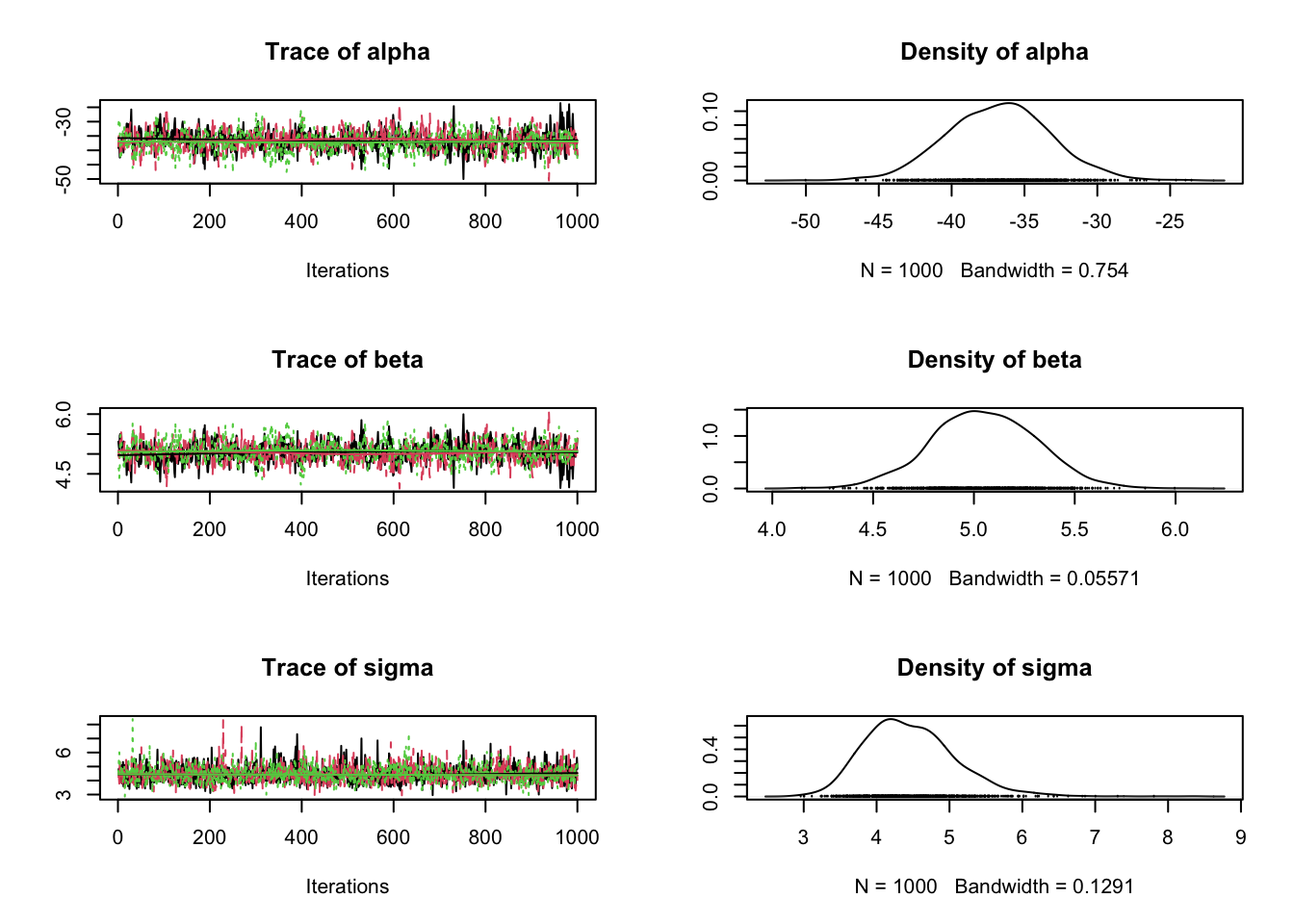plot(analysis1, parm = "beta")plot(analysis1, parm = "sigma")Exercise 4 What is the R-hat value for each of the parameters in the current model with 10,000 iterations?

## jmbr Options

With the the default options_jaggernaut(mode = "report") settings:

• an adaptive phase of 100 iterations is undertaken to maximize sampling efficiency (chain mixing)
• three chains of niters iterations in length are generated.
• the first half of each chain is discarded as burn in.
• each chain os thinned so that the total number of MCMC samples for each monitored parameter is $$\geq 10^3$$.
• convergence is tested under the criterion that $$\hat{R} < 1.1$$
• if convergence has not been achieved the current samples are discarded as burn in and the number of iterations doubled before thinning again.
• this is repeated up to three times or until the convergence threshold of 1.1 is achieved.

To see the current mode and option settings type options_jaggernaut() (or opts_jagr() for short) and for more information type ?set_analysis_mode.

## Chain Mixing

Cross-correlations between parameters, which cause poor chain mixing, i.e., high auto-correlation, can sometimes be eliminated or reduced by reparameterising the model. In the current model, Girth can be centered, i.e., Girth - mean(Girth), in the BUGS code or by setting the select_data argument to be select_data(model1) <- c("Volume", "Girth+").

Exercise 5 What is the effect of centering Girth on the trace plots with 1,000 iterations?

Note if you ever want to examine the actual data being passed to JAGS set the modify_data term of your jags_model object to be a simple function that prints and returns its one argument

modify_data(model1) <- function (data) { print(data); data }

## Derived Parameters

Many researchers estimate fitted values and residuals, generate predictions and perform posterior predictive checks by monitoring additional nodes in their model code.

The disadvantages of this approach are that:

• the model code becomes cluttered.
• the MCMC sampling takes longer.
• adding derived parameters requires a model rerun.
• the table of parameter estimates becomes unwieldy.

jmbr overcomes these problems by allowing derived parameters to be defined in a separate chunk of R code as demonstrated below.

new_expr <- "for(i in 1:length(Volume)) {
prediction[i] <- alpha + beta * Girth[i]

simulated[i] <- rnorm(1, prediction[i], sigma^-2)

D_observed[i] <- log(dnorm(Volume[i], prediction[i], sigma^-2))
D_simulated[i] <- log(dnorm(simulated[i], prediction[i], sigma^-2))
}
residual <- (Volume - prediction) / sigma
discrepancy <- sum(D_observed) - sum(D_simulated)
"

## Predictions

To better understand a model and/or inform management decisions it is generally useful to plot a model’s predictions.

In the following example, the predict function is used to estimate the Volume with 95% CRIs and 95% Prediction Intervals (PRIs) across the range of the observed values of Girth.

prediction <- predict(analysis1, new_data = "Girth", new_expr = new_expr)
simulated <- predict(analysis1, term = "simulated", new_data = "Girth",
new_expr = new_expr)
gp <- ggplot(data = prediction, aes(x = Girth, y = estimate))
gp <- gp + geom_point(data = data_set(analysis1), aes(y = Volume))
gp <- gp + geom_line()
gp <- gp + geom_line(aes(y = lower), linetype = "dashed")
gp <- gp + geom_line(aes(y = upper), linetype = "dashed")
gp <- gp + geom_line(data = simulated, aes(y = lower), linetype = "dotted")
gp <- gp + geom_line(data = simulated, aes(y = upper), linetype = "dotted")
gp <- gp + scale_y_continuous(name = "Volume")

print(gp)

Exercise 6 The new_data argument in the predict function can also take a data.frame. What is the 95% Prediction Interval for the Volume of a tree of Girth 8?

## Residuals

The model assumes that the error terms are independently drawn from a normal distribution. It is possible to assess the adequacy of this assumption by plotting the residual variation.

fitted <- fitted(analysis1, new_expr = new_expr)
fitted$residual <- residuals(analysis1, new_expr = new_expr)$estimate

qplot(estimate, residual, data = fitted) + geom_hline(yintercept = 0) +
geom_smooth(se = FALSE)

Exercise 7 What does the current residual plot suggest to you about model adequacy?

## Posterior Predictive Checks

A complementary approach to assessing model adequacy is to simulate data given the model parameters and compare it to the observed data. Any systematic differences indicate potential failings of the model.

predictive_check(analysis1, derived_code = derived_code)
##             estimate  lower upper   sd error significance
## discrepancy   0.5787 -10.27 12.39 5.65  1958       0.9042

Exercise 8 What does the posterior predictive check suggest to you about model adequacy?

## Allometry

As discussed in the R course notes the relationship between Volume and Girth is expected to be allometric because the cross-sectional area at an given point scales to the square of the girth (circumference).

Expressed as an allometric relationship the model becomes

$Volume_{i} = \alpha * Girth_{i}^\beta * \epsilon_{i}$

which can be reparameterised as a linear regression by log transforming Volume and Girth.

$log(Volume_{i}) = \alpha + \beta * log(Girth_{i}) + \epsilon_{i}$

Variables can be log transformed in the model code or in the select_data argument, i.e., select_data(model1) <- c("log(Volume)", "log(Girth)+").

Exercise 9 Fit the linear regression of the allometric model to the trees data set. Is the model fit improved?

Exercise 10 Is there any support for adding log(Height)+ to the model?

## Significance Values

The significance value in the jaggernaut table of coefficients is twice the probability that the posterior distribution spans zero. As such it represents the Bayesian equivalent of a frequentist two-sided p-value [@greenland_living_2013]. By definition, parameters that represent standard deviations will have a significance value of 0 (as they must be greater than zero).

## Error Values

The error value in the table of coefficients is the percent relative error, which is half the credible interval as a percent of the point estimate. Standard deviations with a uniform prior distribution that is not updated by the data have error values of 0.95. As a general rule, I question the informativeness of parameters representing standard deviations which have an error value $$\geq 0.8$$.

# Tooth Growth

The basic ANCOVA (analysis of covariance) model includes one categorical and one continous predictor variable without interactions. It can be expressed algebraically as follows

$y_{ij} = \alpha_{i} + \beta * x_{j} + \epsilon_{ij}$

where $$\alpha_{i}$$ is the intercept for the ith group mean and $$\beta$$ is the slope and the error terms ($$\epsilon_{ij}$$) are independently drawn from a normal distribution with standard deviation $$\sigma$$.

The following code fits the basic ANCOVA model to the ToothGrowth data and plots the model’s predictions and residuals.

model1 <- jags_model("model {
for(i in 1:nsupp) {
alpha[i] ~ dnorm(0, 40^-2)
}
beta ~ dnorm(0, 20^-2)
sigma ~ dunif(0, 20)

for(i in 1:length(len)) {
eLen[i] <- alpha[supp[i]] + beta * dose[i]
len[i] ~ dnorm(eLen[i], sigma^-2)
}
}",
derived_code  = " data{
for(i in 1:length(len)) {
prediction[i] <- alpha[supp[i]] + beta * dose[i]
}
residual <- (len - prediction) / sigma
}")
data(ToothGrowth)
analysis1 <- jags_analysis(model1, data = ToothGrowth)
coef(analysis1)
##          estimate lower  upper     sd error significance
## alpha    9.307 6.742 11.968 1.3750    28            0
## alpha    5.627 2.989  8.258 1.3549    47            0
## beta        9.730 7.911 11.538 0.9281    19            0
## sigma       4.326 3.625  5.221 0.4004    18            0
prediction <- predict(analysis1, newdata = c("supp", "dose"))

gp <- ggplot(data = prediction, aes(x = dose, y = estimate, color = supp,
shape = supp))
gp <- gp + geom_point(data = dataset(analysis1), aes(y = len))
gp <- gp + geom_line()
gp <- gp + scale_y_continuous(name = "len")

print(gp)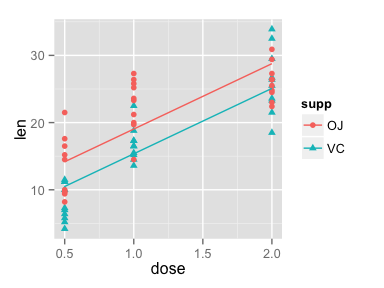residuals <- residuals(analysis1)
residuals$fitted <- fitted(analysis1)$estimate

qplot(fitted, estimate, color = supp, shape = supp, data = residuals, xlab = "Fitted",
ylab = "Residual") + geom_hline(yintercept = 0)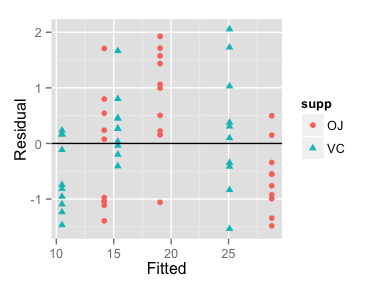Exercise 11 What is the significance value for the effect of OJ versus VC?

Exercise 12 What does the residual plot suggest about the model fit?

## Multiple Models

The linear regression on dose is given by

model2 <- jags_model("model {
alpha ~ dnorm(0, 40^-2)
beta ~ dnorm(0, 20^-2)
sigma ~ dunif(0, 20)

for(i in 1:length(len)) {
eLen[i] <- alpha + beta * dose[i]
len[i] ~ dnorm(eLen[i], sigma^-2)
}
}",
derived_code  = " data{
for(i in 1:length(len)) {
prediction[i] <- alpha + beta * dose[i]
}
residual <- (len - prediction) / sigma
}",
select_data = c("len", "dose"))

The combine function allows multiple jags_model objects which can have unique mode_id’s to be combined into a single object.

model_id(model1) <- "ANCOVA"
model_id(model2) <- "regression"

models <- combine(model1, model2)
analyses <- jags_analysis(models, data = ToothGrowth)
coef(analyses)
## $ANCOVA ## estimate lower upper sd error significance ## alpha 9.218 6.767 11.761 1.2754 27 0 ## alpha 5.540 3.032 8.176 1.2861 46 0 ## beta 9.799 8.109 11.512 0.8637 17 0 ## sigma 4.315 3.623 5.227 0.4192 19 0 ## ##$regression
##       estimate lower  upper     sd error significance
## alpha    7.258 4.867  9.884 1.2902    35            0
## beta     9.889 7.986 11.666 0.9691    19            0
## sigma    4.716 3.890  5.702 0.4601    19            0
prediction <- predict(analyses, newdata = c("supp", "dose"), model_id = "regression")

gp <- gp %+% prediction

print(gp)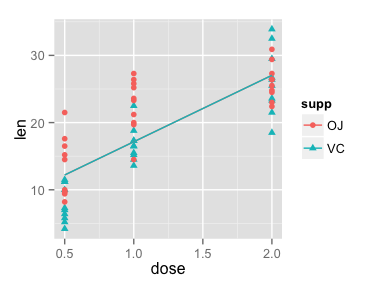Exercise 13 Fit 1) the ANCOVA, 2) the linear regression, 3) the ANOVA and 4) the ANCOVA with an interaction between dose and supp models and plot their predictions. Which model do you prefer?

Exercise 14 How might you further improve your preferred model?

### Effects Size

Often the results of an analysis are easier to understand when they are presented in terms of the percent change in the response [@bradford_using_2005]. The following code predicts and plots the percent change in len relative to 0.75 mg of Vitamin C.

prediction <- predict(analysis1, newdata = c("supp", "dose"), base = data.frame(supp = "OJ",
dose = 0.75))

gp <- ggplot(data = prediction, aes(x = dose, y = estimate, color = supp,
shape = supp))
gp <- gp + geom_line()
gp <- gp + scale_y_continuous(name = "Effect on len (%)", labels = percent)

print(gp)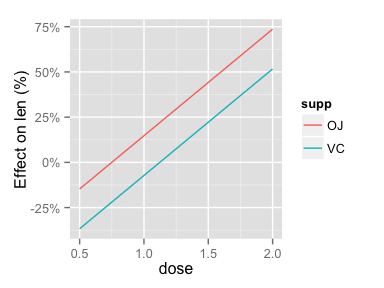Exercise 15 Plot the percent change in len for all four models relative to 0.5 mg of Vitamin C

# Peregrine Falcon Population Abundance

Consider the peregrine falcon population data from Kery and Schuab [-@kery_bayesian_2011]. The following code regresses the number of reproductive Pairs on Year.

model1 <- jags_model("model {
alpha ~ dnorm(0, 100^-2)
beta ~ dnorm(0, 100^-2)
sigma ~ dunif(0, 100)
for(i in 1:length(Pairs)) {
ePairs[i] <- alpha + beta * Year[i]
Pairs[i] ~ dnorm(ePairs[i], sigma^-2)
}
}",
derived_code = "data {
for(i in 1:length(Pairs)) {
prediction[i] <- alpha + beta * Year[i]
}
}",
select_data = c("Pairs", "Year+"))
data(peregrine)

analysis1 <- jags_analysis(model1, data = peregrine)
coef(analysis1)
##       estimate  lower  upper     sd error significance
## alpha   90.565 84.694 96.606 3.0722     7            0
## beta     4.728  4.188  5.244 0.2726    11            0
## sigma   19.430 15.591 23.981 2.1940    22            0
prediction <- predict(analysis1)
gp <- ggplot(data = prediction, aes(x = Year, y = estimate))
gp <- gp + geom_point(data = dataset(analysis1), aes(y = Pairs))
gp <- gp + geom_line()
gp <- gp + geom_line(aes(y = lower), linetype = "dashed")
gp <- gp + geom_line(aes(y = upper), linetype = "dashed")
gp <- gp + scale_x_continuous(name = "Year")
gp <- gp + scale_y_continuous(name = "Pairs")
gp <- gp + expand_limits(y = 0)

print(gp)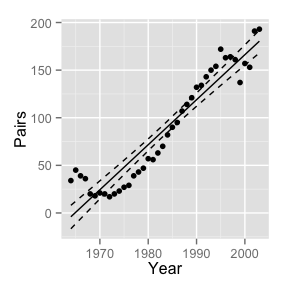Exercise 16 What happens to the model’s predictions if Year isn’t centred?

## Poisson Distribution

The Poisson distribution models counts about a positive mean expected value. It can only assume discrete non-negative values and has a variance equal to the mean.

Exercise 18 Next, replace Pairs[i] ~ dnorm(ePairs[i], sigma^-2) with Pairs[i] ~ dpois(ePairs[i]). How does the assumption of Poisson distributed counts alter the model?

## Polynomial Regression

Curvature in a predictor variable can be modelled by allowing the influence of a variable to vary with the variable. For example, the following model code fits a second-order (quadratic) polynomial regression on Year.

model_code <- "model {
alpha ~ dnorm(0, 100^-2)
beta ~ dnorm(0, 100^-2)
beta2 ~ dnorm(0, 100^-2)
for(i in 1:length(Pairs)) {
log(ePairs[i]) <- alpha + beta * Year[i]  + beta2 * Year[i]^2
Pairs[i] ~ dpois(ePairs[i])
}
}"

Exercise 19 How does the second-order polynomial change the model’s predictions?

Exercise 20 Fit a third-order (cubic) polynomial regression (... + Year[i]^2 + beta3 * Year[i]^3). How does it alter the model?

Exercise 21 Use the third-order polynomial regression to predict the number of breeding pairs in 2006. How confident are you in your answer?

## State-Space Population Growth Model

An alternative to a polynomial regression would be to fit a state-space population growth model which explicity estimates the step changes in the underlying population

$log(N_{t+1}) = log(N_{t}) + r_{t}$ $r_{t} \sim dnorm(\bar{r}, \sigma_{r})$

model6 <- jags_model("model {
mean_r ~ dnorm(0, 1^-2)
sd_r ~ dunif(0, 1)

logN ~ dnorm(0, 10^-2)
for(i in 2:nYear) {
r[i-1] ~ dnorm(mean_r, sd_r^-2)
logN[i] <- logN[i-1] + r[i-1]
}
for(i in 1:length(Pairs)) {
Pairs[i] ~ dpois(exp(logN[Year[i]]))
}
logN1 <- logN
}",
derived_code = "data {
for(i in 1:length(Pairs)) {
log(prediction[i]) <- logN[Year[i]]
}
}",
select_data = c("Pairs", "Year"),
random_effects = list(r = "Year", logN = "Year"))

The specification of r and logN as random effect means that by default they are excluded from the trace plots and table of coefficients.

peregrine$Year <- factor(peregrine$Year)
analysis6 <- jags_analysis(model6, data = peregrine)
coef(analysis6)
prediction <- predict(analysis6)

gp <- gp + aes(x = as.integer(as.character(Year)))
gp <- gp %+% prediction

print(gp)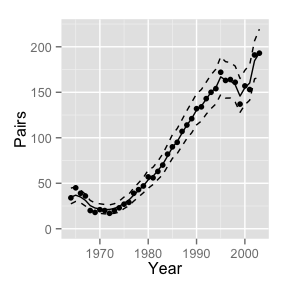Exercise 22 Plot the state-space population growth rate model predictions in terms of the percent change since 1970. What is the estimated percent change in the population in 2003 compared to 1970?

Exercise 23 What is the probability that the population in 2008 will be less than that in 2003? Note you can produce the projections by simply appending five years of missing counts to the dataset.

## Overdispersed Poisson

The Poisson distribution assumes that the variation in the counts about the expected value is due to independent events. Often however this assumption is violated and the variance is greater than the mean. In this case an overdispersed Poisson distribution is required.

The following model uses a gamma distribution where the scale and shape parameters are both 1 / sDispersion^2 to generate the extra-Poisson multiplier because such a distribution has a mean of 1, a standard deviation of sDispersion and is always positive.

model7 <- jags_model("model {
mean_r ~ dnorm(0, 1^-2)
sd_r ~ dunif(0, 1)

logN ~ dnorm(0, 10^-2)
for(i in 2:nYear) {
r[i-1] ~ dnorm(mean_r, sd_r^-2)
logN[i] <- logN[i-1] + r[i-1]
}
sDispersion ~ dunif(0, 5)
for(i in 1:length(Pairs)) {
eDispersion[i] ~ dgamma(1 / sDispersion^2, 1 / sDispersion^2)

Pairs[i] ~ dpois(exp(logN[Year[i]]) * eDispersion[i])
}
logN1 <- logN
}",
derived_code = "data {
for(i in 1:length(Pairs)) {
log(prediction[i]) <- logN[Year[i]]
}
}",
select_data = c("Pairs", "Year"),
random_effects = list(r = "Year", logN = "Year"))
analysis7 <- jags_analysis(model7, data = peregrine)
coef(analysis7)
##             estimate      lower   upper      sd error significance
## logN1        3.54960  3.3016252 3.78656 0.12538     7       0.0000
## mean_r       0.04292 -0.0004407 0.08252 0.02068    97       0.0547
## sDispersion  0.02684  0.0017635 0.07634 0.02063   139       0.0000
## sd_r         0.12741  0.0888883 0.17948 0.02361    36       0.0000
prediction <- predict(analysis7)

gp <- gp %+% prediction

print(gp)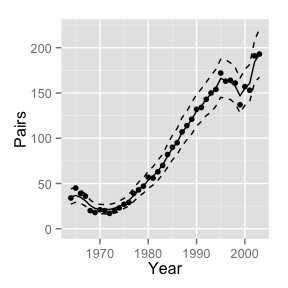Exercise 24 Is the inclusion of a Plot the state-space population growth rate model predictions in terms of the percent change since 1970. What is the estimated percent change in the population in 2003 compared to 1970?

# Peregrine Falcon Breeding Success

Now let us consider the proportion of peregrine Pairs that successfully reproduced (R.pairs/Pairs).

data(peregrine)

peregrine$Proportion <- peregrine$R.Pairs / peregrine$Pairs model1 <- jags_model("model { alpha ~ dnorm(0, 1^-2) beta ~ dnorm(0, 1^-2) sigma ~ dunif(0, 1) for(i in 1:length(Proportion)) { eProportion[i] <- alpha + beta * Year[i] Proportion[i] ~ dnorm(eProportion[i], sigma^-2) } }", derived_code = "data { for(i in 1:length(Proportion)) { prediction[i] <- alpha + beta * Year[i] } }", select_data = c("Proportion", "Year+")) analysis1 <- jags_analysis(model1, data = peregrine) prediction <- predict(analysis1) gp <- ggplot(data = prediction, aes(x = Year, y = estimate)) gp <- gp + geom_point(data = dataset(analysis1), aes(y = Proportion)) gp <- gp + geom_line() gp <- gp + geom_line(aes(y = lower), linetype = "dashed") gp <- gp + geom_line(aes(y = upper), linetype = "dashed") gp <- gp + scale_x_continuous(name = "Year") gp <- gp + scale_y_continuous(name = "Pairs") gp <- gp + expand_limits(y = c(0, 1)) print(gp)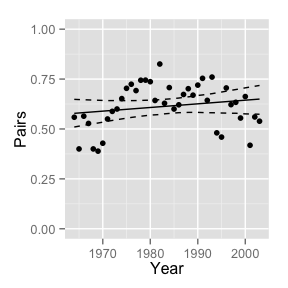Exercise 25 How does a second-order polynomial regression alter the model’s predictions? ## Binomial Distribution The binomial distribution models the number of successes given the expected probability of successes and the number of independent trials. Exercise 27 How does replacing the normal distribution Proportion[i] ~ dnorm(eProportion[i], sigma^-2) with the binomial distribution R.Pairs ~ dbin(eProportion[i], Pairs[i]) alter the model? ## Autoregressive Smoothing Earlier we used an autoregressive term to explicitly model population change. Autoregressive models can also be used simply for their smoothing properties. Here we model the differences in the log-odds probability of breeding success from one year to the next as a normally distributed random effect. model2 <- jags_model("model { theta ~ dnorm(0, 2^-2) sigma ~ dunif(0, 2) for(i in 2:length(R.Pairs)) { theta[i] ~ dnorm(theta[i-1], sigma^-2) } for(i in 1:length(R.Pairs)) { logit(eProportion[i]) <- theta[i] R.Pairs[i] ~ dbin(eProportion[i], Pairs[i]) } }", derived_code = "data { for(i in 1:length(R.Pairs)) { logit(prediction[i]) <- theta[Year[i]] } }", random_effect = list(theta = "Year"), select_data = c("R.Pairs", "Pairs", "Year")) data(peregrine) peregrine$Year <- factor(peregrine\$Year)

analysis2 <- jags_analysis(model2, data = peregrine)
prediction <- predict(analysis2)

gp <- gp + aes(as.integer(as.character(Year)))
gp <- gp %+% prediction

print(gp)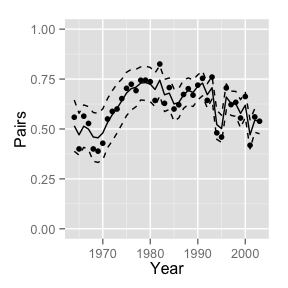Exercise 28 What happens to the model if you replace line 5 with theta[i] <- theta[i-1]?

## Overdispersed Binomial Distribution

The binomial distribution assumes independent trials. Often this is not the case. Overdispersion can be simply modelled through a random effect on the expected probability of success for each sample.

Exercise 29 What effect does a normally distributed random effect on logit(eProportion[i]) have on the model? Are the data overdispersed?

# Further Information

The JAGS manual is definitely worth a read to familiarise yourself with the available distributions and functions.